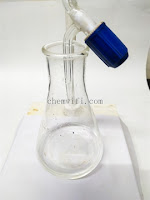# How to prepare & standardization 0.1 N Hydrochloric acid

## 0.1 N Hydrochloric Acid ( HCl )

### Preparation ) 0.1 N Hydrochloric Acid Solution

• Take 8.3 ml of concentrated hydrochloric acid (Sp gr 1.19) into a 1000-ml volumetric flask.
• Dilute to the mark with water, mix well, and
• Store in a tightly closed glass container.

### I. Standardization  0.1 N Hydrochloric Acid with Sodium Carbonate

• Take 0.5 oto 1 g of primary standard anhydrouso sdium carbonate (Na2CO3) to a suitable dish or crucible, and dry at 250°C for 4 h.
• Cool in a desiccator.
• Weight accurately 0.22 g of the dried Na2CO3, and transfer to a 250-mL conical flask.
• Add 50 mL of water, swirl to dissolve the carbonate, and add 2 drops of a 0.1 % solution of methyl red in alcohol.
• Titrate with the HCl solution.
Initial point color     End point color#### Calculation

Normality of the Hcl solution(N), as
follows:
N = A /0.053*B

Where
A - gram of Na2CO3 used,
B - used ml of Hcl solution in titrition.

Ex-
0.2203 gm of Na2CO3
41.7 ml used of Hcl solution.

Normality of the Hcl= 0.2203/0.053*41.7= 0.0997 N

#### Stability

Re-standardize monthly.

#### II. Standardization with Tris(Hydroxymethyl)- Amimethane

• Take 1 to 2 g of primary standard Tris (hydroxymethyl) Aminomethane [(HOCH2)3CNH2] to a suitable dish or crucible, and dry in a vacuum at 70°C for 24 h.
• Cool in a desiccator.
• Weigh accurately 0.40 g of the dried tris(hydroxymethyl)aminomethane, and transferto a 250-ml conical flask.
• Dissolve in 50 ml of ammonia- and carbon dioxide-free water, and titrate with the HCl solution to a pH of 4.70 using a suitable pH meter.

#### Calculation

Normality of the Hcl solution(N), as
follows:
N = A /0.1211*B
Where
A - gram of Tris(Hydroxymethyl)- Amimethane used,
B - used ml of Hcl solution in titrition.
Ex-
0.4002 gm of Tris(Hydroxymethyl)- Amimethane
33.1 ml used of Hcl solution.
Normality of the Hcl= 0.4002/0.1211*33.1= 0.0998N

#### Stability

Re-standardize monthly.